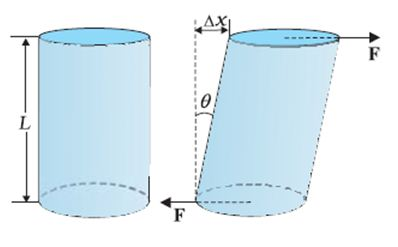# Topic: Mechanical Properties of Solids (Test 1)

Topic: Mechanical Properties of Solids
Q.1
A perfectly rigid body is one
A. whose shape and size do not change on application of force
B. whose shape and size change on application of force
C. which starts flowing like water on application of force
D. which does not move on application of force
Answer : Option A
Explaination / Solution:

A perfectly rigid body is hypothetical in nature but for some phenomena ( in rotational bodies ) we assume bodies are perfectly rigid i.e. the intermolecular forces are always in equilibrium irrespective of the external forces due to which their shape and size are constant.

Workspace
Report
Q.2
Elasticity is the property of a body, by virtue of which
A. it is distorted or stretches without the application of force
B. it changes size and shape when the force is applied and stays in that shape when applied force is removed
C. it tends to regain its original size and shape when the applied force is removed
D. it remains in original size and shape when the force is applied
Answer : Option C
Explaination / Solution:

When external force is applied on the solid bodies, the solid bodies get deformed. The atoms or molecules are displaced from their equilibrium positions causing a change in the interatomic ( or intermolicular ) distances. When the deforming foce is removed, the interatomic forces tend to drive them back to their original postions. Thus the body regains its original shape and size.

Workspace
Report
Q.3
The importance of the elastic behavior of materials is
A. that it is useful in making springs

B. that it gives methods for understanding materials
C. that it is useful in building sling shots
D. that it enables a safe and sound design of bridges, buildings, machinery parts.
Answer : Option D
Explaination / Solution:

More the elastic a material is , more it has the property to regain its original position which is required in construction works.

Workspace
Report
Q.4
When a solid is deformed,
A. all the atoms or molecules are displaced from their equilibrium positions causing a change in inter atomic (or intermolecular) distances.
B. only the atoms or molecules at some points move from their equilibrium position
C. the atoms or molecules do not move from their equilibrium position
D. only the atoms or molecules of the surface move from their equilibrium position
Answer : Option A
Explaination / Solution:

External force permanently distubed the equilibrium position of the interatomic ( or intermolecular ) forces between the particles of solid bodies.

Workspace
Report
Q.5
Stress is
A. force per unit length
B. force per unit area
C. total applied force
D. three point average of forces
Answer : Option B
Explaination / Solution:
No Explaination.

Workspace
Report
Q.6
The S.I unit of stress is
A. Pascal
B. Joule
C. Newton
D. Watt
Answer : Option A
Explaination / Solution:
No Explaination.

Workspace
Report
Q.7
If a wire has an initial length L and becomes L +  on application of force, the longitudinal strain is given by

A. ΔL /(3L)
B. ΔL /L
C. ΔL
D. ΔL /(2L)
Answer : Option B
Explaination / Solution:
No Explaination.

Workspace
Report
Q.8
Referring to figure shearing strain is defined as.A. the ratio of relative displacement of the faces Δx to the length of the cylinder L
B. cosθ
C. sinθ
D. cotθ
Answer : Option A
Explaination / Solution:
No Explaination.

Workspace
Report
Q.9
volume strain is defined
A. as the ratio of change in volume (ΔV) to thrice the original volume V
B. as the change in volume ΔV
C. as the ratio of change in volume (ΔV) to twice the original volume V
D. as the ratio of change in volume (ΔV) to the original volume V
Answer : Option D
Explaination / Solution:
No Explaination.

Workspace
Report
Q.10
According to Hooke’s law
A. For small deformations the stress is proportional to square of strain
B. For large deformations the stress and strain are proportional to each other
C. For small deformations the stress and strain are proportional to each other
D. For small deformations the stress and strain are inversely proportional to each other
Answer : Option C
Explaination / Solution:
No Explaination.

Workspace
Report